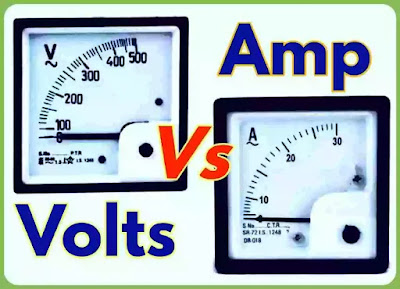--> Difference between Voltage and Ampere (Volts vs Amp)

## \$type=carousel\$clm=4

Volt is SI unit of voltage and EMF that drives electrons to flow through conductor, Whereas Amp is SI unit of current which is rate of flow of charge.

Actually Volts and Ampere are two different SI units used in electrical engineering for the measurement of Voltage and electric current. The main difference between Volts and Ampere (volts vs Amp) is: Volt is the SI unit of measuring Potential difference between two points, Voltage, and EMF (Electromotive force) Whereas Ampere or Amp is the SI unit of measuring current flowing in the circuit.

In this article, At first we are going to understand that what is Volt and Ampere?, then after that we will discuss our main topic " Difference between Volts and Ampere or (Volts vs Amp)". So let's start the topic.

## What is Volt?

Volt is the SI unit of measuring Electromotive force (EMF) and potential difference (Voltage) between two points. Electromotive force (EMF) is the force that drives electrons to flow through the conductor, Whereas electric potential difference or voltage is: When you connect voltmeter across two terminal of battery then what voltmeter reads is called potential difference or voltage.

Since both voltage and EMF is measured by voltmeter in terms of Volt. So we can say that volt is the SI unit of EMF and Voltage. It is represented by a capital alphabet V and measured by voltmeter.

## What is Ampere or Amp?

Ampere or Amp is the SI unit of measuring current in electrical circuit. It measures the rate of flow of electric charge flowing through any conductor. It is measured by Ammeter in terms of Amp or Ampere and represented by capital alphabet A.

1 Ampere is equal to 1 coulomb of charge flowing through conductor in 1 second. Mathematically, It is 6.242 * 1018 times of elementary charges.

[ ##eye## Working of Star-Delta starter]

Now, let's come to our main topic " Difference between Voltage and Ampere or (Volts vs Ampere)".

## Difference between Voltage and Ampere (Volts vs Amp)

In electrical engineering, Volt and Ampere both are the units of two different electrical quantities i.e, Voltage and electric current respectively. So there are many differences between them. Let's understand it with these points.

• Volt is the measure of force (EMF) that drives electrons to flow through the conductor, Whereas Ampere is the measure of rate of flow of charge flowing through the conductor.

• Volt is SI unit of EMF and Voltage, Whereas Ampere is the SI unit of electric current.

• Volt is represented by Joule per Coulomb, Whereas Amp is represented by Coulomb per second.

• Volt is measured by voltmeter, Whereas Amp is measured by Ammeter.

• Volt is denoted by V, Whereas Ampere is denoted by A.

### Comparison chart of Volts vs Amp.

Properties Volts Ampere
Definition Volt is unit to measure voltage and EMF Ampere is unit to measure current
Formula Joule/coulomb Coulomb/second
Abbreviation Volt is denoted by V Ampere is denoted by A
Measuring Quantity Voltage, EMF and Potential difference Electric Current
Measuring Instrument Voltmeter Ammeter
Analogy It is analogous to pressure of water stored in pipe It is analogous to the rate or speed of water flow through pipe

## Volts vs Amp: Key points

Volts:

• It is the measurement unit of EMF and Potential difference.
• It is denoted by V.
• It is measured with the help of voltmeter in parallel across two points in the circuit.
• It is defined as Joule per Coulomb. i.e, Volt = Joule/Coulomb
• Hence 1 volt is defined as: When 1 joule work is done in carrying charge of 1 coulomb from infinity to a point in the electric field, then potential at that point is 1 volt.

Ampere:

• It is the measurement unit of electric current.
• It is denoted by A.
• It is measured with the help of Ammeter connected in series in the circuit.
• It is defined as Coulomb per sec. i.e, Ampere = Coulomb/Second or A = C/S.Name

Digital Electronics,1,Electrical Basics,15,Electrical Machine,4,Electrical Q & A,2,Electronics,2,Guest Post,3,Instrumentation,4,Mathematics,4,Power Factor,3,Power System,10,
ltr
item
Electrical-Technology: Difference between Voltage and Ampere (Volts vs Amp)
Difference between Voltage and Ampere (Volts vs Amp)
Volt is SI unit of voltage and EMF that drives electrons to flow through conductor, Whereas Amp is SI unit of current which is rate of flow of charge.